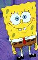Math Is Fun Forum

Discussion about math, puzzles, games and fun.   Useful symbols: ÷ × ½ √ ∞ ≠ ≤ ≥ ≈ ⇒ ± ∈ Δ θ ∴ ∑ ∫  π  -¹ ² ³ °

You are not logged in.

#1 2019-11-12 22:55:16

George,Y
MemberRegistered: 2006-03-12
Posts: 1,379

Cross Redundency Reliability Probability

I designed a server sytem and its backup plan.

To simplify, there are a pair of server programs  each using one hard server.
And redundancy makes a pair of them.

This is the original plan:

A1 A2
|    |
B1 B2

But since A's are equivalent and B's are equivalent I come up this:

A1 A2
|  X  |
B1 B2

A1-B2 and A2-B1 also works.

Now comes the fun part: reliability
Suppose the manufacturer can only guarantee the failure rate of each hard server as little as p

How is the overall A-B server system failure rate?

For the straight pair, the failure situation is not a single pair could work (They both fail)
[1-(1-p)(1-p)]^2 = [p(2-p)]^2

For the cross enhanced, the success situation is both A's and B's have at least one functional:
[1-pp]^2

So the survival rate of the straight is S(p) = 1 - [p(2-p)]^2  ; failure rate s(p) = [p(2-p)]^2
the survival rate of cross enhanced is C(p) = [1-pp]^2  ; failure rate c(p) = 1 - (1-pp)^2

Usually, s(p) = 2c(p)
Or the cross pairing reduces the original failure rate by half.

X'(y-Xβ)=0

Offline

#2 2019-11-13 01:57:20

George,Y
MemberRegistered: 2006-03-12
Posts: 1,379

Re: Cross Redundency Reliability Probability

s(p)/c(p) -> 2 as p->0

X'(y-Xβ)=0

Offline